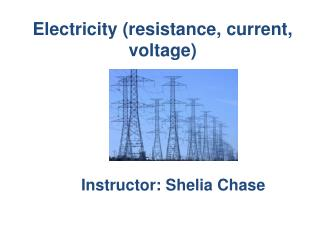DownloadDownload PresentationElectricity (resistance, current, voltage)

# Electricity (resistance, current, voltage)

Télécharger la présentation## Electricity (resistance, current, voltage)

- - - - - - - - - - - - - - - - - - - - - - - - - - - E N D - - - - - - - - - - - - - - - - - - - - - - - - - - -
##### Presentation Transcript

1. Electricity (resistance, current, voltage) Instructor: Shelia Chase

2. Electricity A form of energy resulting from the existence of charged particles (such as electrons or protons), either statically or as an accumulation.

3. Electric Current • The flow of electric current • Charge flows when there is a potential difference, difference in potential (voltage) between ends of a conductor • In solids, electrons carry the flow • In fluids, positive and negative ions as well as electrons may flow

4. Example If one end of a wire were connected to the ground and the other end placed on a Van de Graaff generator that is charged to a high potential, charge would flow through the wire. This would be brief flow, to have longer flow you would need to maintain the potential difference.

5. Potential Difference http://www.youtube.com/watch?v=F1p3fgbDnkY

6. Voltage The voltage source is something that provides the potential difference. If you charge a metal sphere positively, and another negatively, you can develop a large voltage between them. http://www.youtube.com/watch?v=1xPjES-sHwg

7. Resistance The current also depends on the resistance that the conductor offers to the flow of the charge- the electrical resistance.

8. Resistance • Similar to the rate of water flow in a pipe • Thick wires have less resistance than thin wires • Longer wires have more resistance than short wires.

9. Resistance Measured in units called ohms, after Georg Simon Ohm, a German physicist who tested different wires in circuits to see what effect the resistance of the wire had on the current.

10. Ohm’s Law Ohm discovered that the current in a circuit is directly proportional to the voltage impressed across the circuit and is inversely proportional to the resistance of the circuit, current = voltage/resistance

11. Physics is fun!